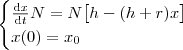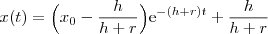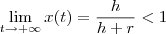My Email: yeshengzou@gmail.com

### 我的博客解决方案（继2）

```import vim,os,markdown2,re,datetime

reInLineMath = re.compile(r'\＄.*?\＄')
reBlockMath = re.compile(r'\\\［.*?\\\］', re.S)

def getFileName():
'get a random filename like 20090423'
d = datetime.date.today().strftime('%Y%m%d')
return d

def createTexFile(body, mathInLineList, mathBlockList):
mathInLineList = '\n\\newpage\n'.join(mathInLineList)
mathBlockList = '\n\\newpage\n'.join(mathBlockList)
texcode = '''
\\documentclass{article}\n
\\usepackage{tikz}\n
\\usepackage{amsmath}\n
\\pagestyle{empty}\n
\\\begin{document}
%s\n\\newpage\n%s\n
\\end{document}''' % (mathInLineList, mathBlockList)
file = open('/home/zys/temp/%s.tex' % getFileName(),'w')
print >> file,texcode
file.close()
return file.name

subject = vim.current.buffer
body = '\n'.join(vim.current.buffer[2:])

mathInLineList = reInLineMath.findall(body)
mathBlockList = reBlockMath.findall(body)

if mathInLineList.__len__() > 0 or mathBlockList.__len__() > 0:

texFile = createTexFile(body, mathInLineList = mathInLineList,
mathBlockList = mathBlockList)
os.system('rxvt -e pdflatex --output-directory=/home/zys/temp/ %s' %
texFile)
os.system('rxvt -e convert -trim  -density 120 -antialias %s.pdf %s.
jpg' % (texFile.split('.'),texFile.split('.')))

mathList = mathInLineList + mathBlockList
if mathList.__len__() == 1:
body = body.replace(mathList[i],
'!［math］(%s.jpg)' % (texFile.split('.')))
else:
for i in range(mathList.__len__()):
body = body.replace(mathList[i],
'!［math］(%s-%d.jpg)' % (texFile.split('.'), i))
```

### 八卦传播问题### 参考

1. evlag said...
你的BOLG变化好大，你学过PYTHON？我也因工作需要要学LUA了。。
ffan said...
是啊，我考虑自适应加无图的页面。
python我自己学来平时用的，好用啊，哈哈。
貌似有很多游戏都用LUA做嵌入式扩展脚本吧。
evlag said...
LUA最出就是WOW嘛。。没想到我现在的公司也要用=。=
PS：你空间变得很想WIKI了。。不太好看
ffan said...
不光是WOW，wiki上看，RO也用，后面应该还有更多的游戏会用它作扩展吧。不过我倒是从来没有接触过它，当初WOW的自定义宏我觉得很强。

我最早听说LUA是TeX的一个引擎luatex，它可以用LUA作扩展。其它还有什么应用就不知道了。